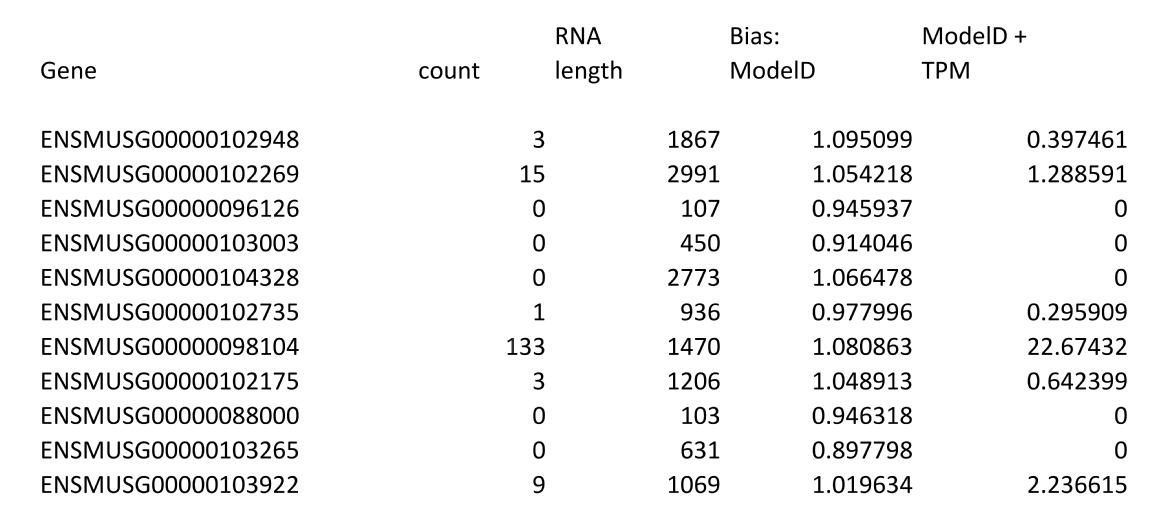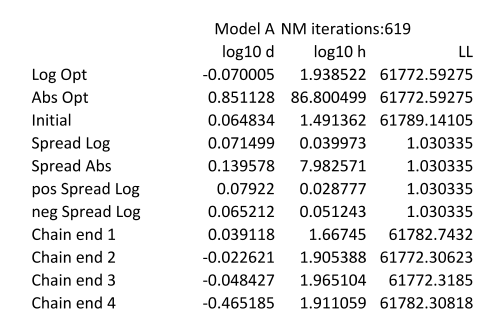# Output files

The default output from LiBiNorm consists of a single text file that lists the expression values for each gene. There are a number of other optional text files that can be produced and which provide more detail about the bias normalisation using -u <fileroot>

## Default gene expression output

By default the only output from LiBiNorm is a list of genes and their expression in text format which has the following format and is sent to stdout, mirroring the operation of htseq-count:The first two columns are identical to those of htseq-count. There then follows the RNA length, the predicted bias relative to a linear model (= reads scale proportional to length, e.g. FPKM) and the expression in TPM after correction for the bias as predicted by the bias model that has been selected.

LiBiNorm can also output the results directly to a file using -c <filename>.

If LiBiNorm is run in the htseq-count compatible mode (-z), then only the first two columns are output and there is no header information.

The full mode option (-f) provides additional columns showing a range of different expression values, with and without bias normalisation.

## Additional output files providing details of the bias analysis

When the -u <fileroot> option is used up to five additional files are created which provide more details on the results of the bias normalisation

<fileroot>_bias.txt

This shows the bias in the underlying read distribution as the raw data for a heat map that can then be plotted using LiBiNormPlot.R. The genes are ordered by transcript length and then grouped into 500 bins that are as equal in size as possible. Within each bin a histogram of the distribution of the reads is calculated, dividing the transcript into 100 equal portions. The output file contains the mean transcript length for each pin followed 100 numbers giving the normalised read distribution for the bin.

<fileroot>_results.txt

This provides the best parameters and associated log likelihoods for the model (or all of the models if the -f option is used), together with an indication of the variation seen within the MCMC chain used to derive the parameters. The endpoints of each of the chains are also listed. The following shows an example for one of the models. The columns show the results for each of the parameters in the model and the (negative) Log Likelihood that is obtained.

The first two rows give the log10 and absolute values for the model parameters. The "Initial" row identifies the values obtained by Nelder Mead that were used as a starting point for the MCMC runs. The next four rows give various measures of the dispersion (median absolute deviation) in the values as determined by the MCMC runs. Only a single measure of the (negative) Log Likelihood is shown. The following rows show the end points of each of the MCMC runs which allow checking agreement between these.NM iterations indicate how many iterations of the Nelder Mead algorthm were run before the parameters had stabilised sufficiently for LiBiNorm to move to the second, MCMC based stage of parameter determination.

<fileroot>_norm.txt

This outputs the predicted bias for RNA lengths between 200 bp and 20000 bp for each of the 6 models. Parameter estimates are given for each model, followed by two rows indicating the predicted bias at 100 bp intervals between 100 and 20000 bp. A length of 1000 bp is used as the reference.

<fileroot>_expression.txt

This file mirrors the counts data that is produced by LiBiNorm unless the -j option is used in which case it provides a complete set of raw and bias corrected TPM and RKPM figures for each gene.

<fileroot>_distribution.txt

Thhis is similar to the <fileroot>_bias.txt file except that only 11 bins are used, centered on transcript lengths distributed pseudo logarithmically between 200 and 20000 bases. The read distribution is again calculated for each bin. Because the bins are much larger this generates a much smoother indication of the read distribution. The file also contains the read distribution predicted by one (or all if the -f option is used) of the models.

The file is divided into two sections. The first only contains information for 5 of the transcript length bins and it is arranged in a form that is suitable for plotting with R. The second section repeats the data, but this time including the values for all 11 bins, presented in a form that is more suitable for use in a spreadsheet.